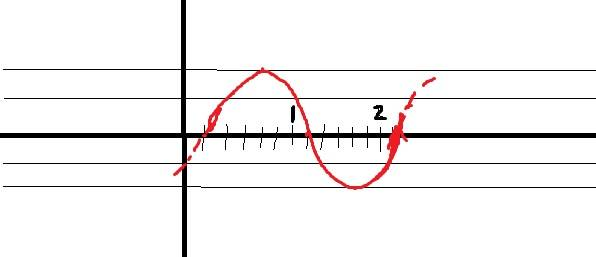# Determine the equation when given a graph

## Homework StatementThe graph goes on for ever in both directions and we know that it's in the form
y=Asin(Bx+C)

## The Attempt at a Solution

I'm not sure what to do here. The amplitude is easy, it's 2.

C/B = Period
Correct?

I'm at a loss here.

Mark44
Mentor

## Homework StatementThe graph goes on for ever in both directions and we know that it's in the form
y=Asin(Bx+C)
Or y = A sin(B(x + C/B))

## The Attempt at a Solution

I'm not sure what to do here. The amplitude is easy, it's 2.

C/B = Period
Correct?
No. If you had y = sin(x + D), the period would be ##2\pi##.
If you had y = sin(2(x + D)), the period would be ##2\pi/2##, right?

I'm at a loss here.

Hmm I'm not sure I'm following your logic here. What throws me off is the fact that I have no pies on the X-axis. I have no idea how to start calculating the period.

If I had a 2pi it would be easy enough, I'd just see how many cycles the graph completes in a 2pi interval.

Mark44
Mentor
Hmm I'm not sure I'm following your logic here. What throws me off is the fact that I have no pies on the X-axis. I have no idea how to start calculating the period.
From your graph, can you estimate what the period is?
If I had a 2pi it would be easy enough, I'd just see how many cycles the graph completes in a 2pi interval.

P=12/13

?

Mark44
Mentor
P=12/13

?
???

How did you get that?

Mark44
Mentor
Your graph shows slightly more than one period of this function.

I graphed it by hand since I remembered it of my final today and I couldn't solve it. Assume that the graph goes on forever.

Hmm okay let me retry.
Every integer is divided into 6 parts as you can see from the graph.
When calculating the period I start from the 1/6 and go to 13/6 where the graph repeats itself.
This means that the period is 12/6 = 2?
The phase shift is 1/6 so the end result would be

y=2sin(2x-1/6)

Correct?

I feel like I'm missing a pi somewhere.... Is it y=2sin(pi(x)-1/6) ?

Last edited:
Mark44
Mentor
I graphed it by hand since I remembered it of my final today and I couldn't solve it. Assume that the graph goes on forever.

Hmm okay let me retry.
Every integer is divided into 6 parts as you can see from the graph.
When calculating the period I start from the 1/6 and go to 13/6 where the graph repeats itself.
This means that the period is 12/6 = 2?
Yes.
The phase shift is 1/6 so the end result would be

y=2sin(2x-1/6)

Correct?
No, but you're getting closer than you were before.

Let's look at a simpler example, y = sin(2x). Its period is ##2\pi/2 = \pi##. Your function has a period of 2, so the number that multiplies x needs to be what?
I feel like I'm missing a pi somewhere.... Is it y=2sin(pi(x)-1/6) ?

This is very close. If you want to shift the graph of y = sin(2x) to the right by 1/2, it would be y = sin(2(x - 1/2)). Does that give you an idea?

Hmm... Okay. My period is

2pi / 2 = pi.
y=2sin(pi(x))

Now, to phase shift 1/6 of a unit I do

y = 2sin(pi(x) - pi/6)

?

Dick
Homework Helper
Hmm... Okay. My period is

2pi / 2 = pi.
y=2sin(pi(x))

Now, to phase shift 1/6 of a unit I do

y = 2sin(pi(x) - pi/6)

?

That looks right.

Thanks a bunch. Much appreciated. I totally bombed my final but at least I walked away with some new knowledge.